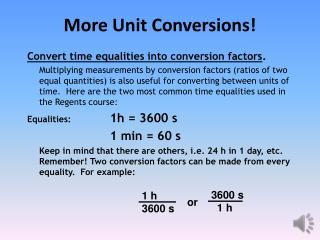DownloadDownload PresentationMore U nit Conversions!

# More U nit Conversions!

Download Presentation## More U nit Conversions!

- - - - - - - - - - - - - - - - - - - - - - - - - - - E N D - - - - - - - - - - - - - - - - - - - - - - - - - - -
##### Presentation Transcript

1. More Unit Conversions! Convert time equalities into conversion factors. Multiplying measurements by conversion factors (ratios of two equal quantities) is also useful for converting between units of time. Here are the two most common time equalities used in the Regents course: Equalities:1h = 3600 s 1 min = 60 s Keep in mind that there are others, i.e. 24 h in 1 day, etc. Remember! Two conversion factors can be made from every equality. For example: 1 h 3600 s 3600 s 1 h or

2. Type 1 Example with time conversion (Prefix to Base) Convert 15 μg/h (micrograms/hour)to g/min(grams/minute). 1 h 15 µg = 15 x10-6 g h 60 min x10-6 = 2.5 x 10-7g/min Do the type one unit conversion first, then multiply by the appropriate conversion factor to convert the time units.

3. Type Two Example with time conversion (Base to prefix) Convert 1500m/h (meters/hour) to km/s (kilometers/second). 1 h 1.5x103 m 1 km 3600 s h 103m = 4.17 x 10-4km/s

4. Type Three Example (Prefix to prefix) Note: Just do a Type 1 on the metric units first, then perform the Type 2 with time conversion as shown on the previous slide.